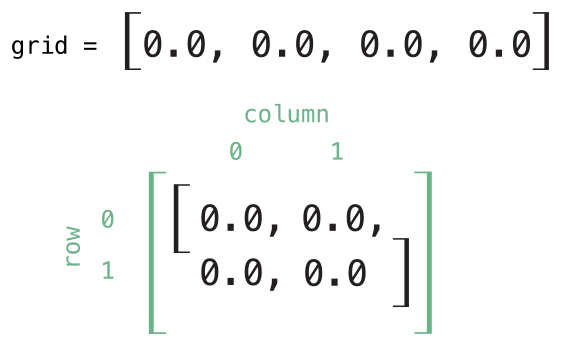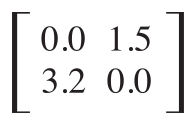# Swift学习之路-下标

## 下标的语法

``````subscript(index: Int) -> Int {
get {
// return an appropriate subscript value here
}
set(newValue) {
// perform a suitable setting action here
}
}``````

newValue 的类型和下标的返回值一样。与计算属性一样，你可以选择不去指定 setter 的( newValue )形式参数。 setter 默认提供形式参数 newValue ，如果你自己没有提供的话。

``````subscript(index: Int) -> Int {
// return an appropriate subscript value here
}``````

``````struct TimesTable {
let multiplier: Int
subscript(index: Int) -> Int {
return multiplier * index
}
}
let threeTimesTable = TimesTable(multiplier: 3)
print("six times three is \(threeTimesTable)")
// prints "six times three is 18"``````

n倍表是基于固定的数学公式。并不适合对 threeTimesTable[someIndex] 进行赋值操作，所以 TimesTable 的下标定义为只读下标。

## 下标用法

“下标”确切的意思取决于它使用的上下文。通常下标是用来访问集合、列表或序列中元素的快捷方式。你可以在你自己特定的类或结构体中自由实现下标来提供合适的功能。

``````var numberOfLegs = ["spider": 8， "ant": 6， "cat": 4]
numberOfLegs["bird"] = 2``````

Swift 的 Dictionary 类型实现它的下标为接收和返回可选型的下标。对于上例中的 numberOfLegs 字典，键值下标接收和返回一个 Int? 类型的值，或者说“可选的 Int  ”。 Dictionary 类型使用可选的下标类型来建模不是所有键都会有值的事实，并且提供了一种通过给键赋值为 nil 来删除对应键的值的方法。

## 下标选项

``````struct Matrix {
let rows: Int, columns: Int
var grid: [Double]
init(rows: Int, columns: Int) {
self.rows = rows
self.columns = columns
grid = Array(repeating: 0.0, count: rows * columns)
}
func indexIsValid(row: Int, column: Int) -> Bool {
return row >= 0 && row < rows && column >= 0 && column < columns
}
subscript(row: Int, column: Int) -> Double {
get {
assert(indexIsValid(row: row, column: column), "Index out of range")
return grid[(row * columns) + column]
}
set {
assert(indexIsValid(row: row, column: column), "Index out of range")
grid[(row * columns) + column] = newValue
}
}
}``````

Matrix 提供了一个接收 rows 和 columns 两个形式参数的初始化器，创建了一个足够容纳 `rows * columns` 个数的 Double 类型数组。矩阵里的每个位置都用 0.0 初始化。要这么做，数组的长度，每一格初始化为 0.0 ，都传入数组初始化器来创建和初始化一个正确长度的数组。这个初始化器在使用默认值创建数组里有更详细的描述。

``var matrix = Matrix(rows: 2, columns: 2)````````matrix[0, 1] = 1.5
matrix[1, 0] = 3.2``````Matrix 下标的设置器和读取器都包含了一个断言来检查下标的 row 和 column 是否有效。为了方便进行断言， Matrix 包含了一个名为 `indexIsValid(row:column:)` 的成员方法，它用来确认请求的 row 或 column 值是否会造成数组越界：

``````func indexIsValidForRow(row: Int, column: Int) -> Bool {
return row >= 0 && row < rows && column >= 0 && column < columns
}``````

``````let someValue = matrix[2, 2]
// this triggers an assert, because [2, 2] is outside of the matrix bounds``````

## 类型下标

``````enum Planet: Int {
case mercury = 1, venus, earth, mars, jupiter, saturn, uranus, neptune
static subscript(n: Int) -> Planet {
return Planet(rawValue: n)!
}
}
let mars = Planet
print(mars)``````